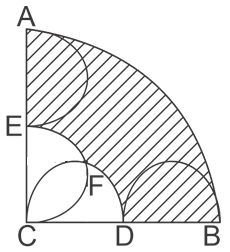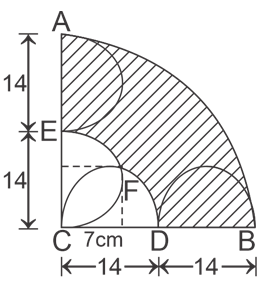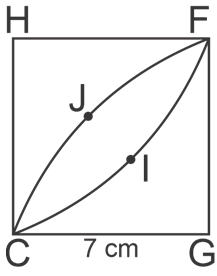Question of The Day17-08-2020

# What will be the area of the shaded region in the figure if the radius of each semicircle is 7 cm?Correct Answer : c ) 490 cm2

Explanation :

Construction: In the given figure, draw two perpendiculars from point F to line CD and CE.Area of common portion CFHI will be = (Area of quarter circle CJFG + Area of quarter circle CIFH) – Area of square

We know,

Area of quadrant CJFG and CIFH =$$\dfrac{1}{4}(π(r)^2)$$ and Area of square CGFH = (side)2

$$⇒Area \: of \: CFHI=\dfrac{1}{4}(π(7)^2)+\dfrac{1}{4}(π(7)^2)-7^2$$

$$Area \:of \:CFHI=\dfrac{49}{4}π+\dfrac{49}{4}π-49$$

$$Area \: of \:CFHI=\dfrac{49}{2}×\dfrac{22}{7}-49=77-49=28 \space cm^2$$

Now, Area of Shaded region will be = Area of quadrant ACB – Area of semicircle EFC – area of semicircle CFD + Area of CFHI

$$⇒\dfrac{1}{4}π(28)^2-\dfrac{1}{2}π(7)^2-\dfrac{1}{2}π(7)^2+28 \space cm^2$$

$$⇒196π-49π+28$$

$$⇒147×\dfrac{22}{7}+28=462+28=490 \space cm^2$$

Hence, (c) is the correct answer.

Questions related to Mensuration are asked in various government exams like SSC CGL, SSC MTS, SSC CPO, SSC CHSL, RRB JE, RRB NTPC, RRB GROUP D, RRB OFFICER SCALE-I, IBPS PO, IBPS SO, RRB Office Assistant, IBPS Clerk, RBI Assistant, IBPS RRB OFFICER SCALE 2&3, UPSC CDS, UPSC NDA, UP SI etc.

Read Daily Current Affairs, Banking Awareness, Hindi Current Affairs, Word of the Day, and attempt free mock tests at PendulumEdu and boost your preparation for the actual exam.0